# Understanding BEMT source code

Dear @Jason.Jonkman

Could you please help me what is “phiIn” in the subroutine copied below. The code is part of the OpenFAST source code BEMT module. Is the phiIn = atan(Vx/Vy) because induction factors are not computed yet, i mean when this is called. so is it the angle calculated without multiplying induction factors a, a’ to Vx and Vy respectively?

subroutine GetSolveRegionOrdering(Vx, phiIn, test_lower, test_upper)
** real(ReKi), intent(in ) :: Vx**
** real(ReKi), intent(in ) :: phiIn**
** real(ReKi), intent( out) :: test_lower(3)**
** real(ReKi), intent( out) :: test_upper(3)**

** if (Vx > 0) then**

** test_lower(1) = BEMT_epsilon2**
** test_upper(1) = PiBy2 - BEMT_epsilon2**

** if (phiIn < pi/4.0_ReKi .and. phiIn > -pi/4.0_ReKi) then !bjj: added the negative for those cases where the previously calculated non-BEMT phi is in the [-pi,-pi/4] range**
** test_lower(2) = -pi/4.0_ReKi**
** test_upper(2) = -BEMT_epsilon2**

** test_lower(3) = PiBy2 + BEMT_epsilon2**
** test_upper(3) = pi - BEMT_epsilon2**
** else**
** test_lower(3) = -pi/4.0_ReKi**
** test_upper(3) = -BEMT_epsilon2**

** test_lower(2) = PiBy2 + BEMT_epsilon2**
** test_upper(2) = pi - BEMT_epsilon2**
** end if**

** else**

** test_lower(1) = -BEMT_epsilon2**
** test_upper(1) = -PiBy2 + BEMT_epsilon2**

** if (phiIn > -pi/4.0_ReKi .and. phiIn < pi/4.0_ReKi) then !bjj: added the negative for those cases where the previously calculated non-BEMT phi is in the [-pi,-pi/4] range**
** test_lower(2) = pi/4.0_ReKi**
** test_upper(2) = BEMT_epsilon2**

** test_lower(3) = -PiBy2 - BEMT_epsilon2**
** test_upper(3) = -pi + BEMT_epsilon2**
** else**
** test_lower(3) = pi/4.0_ReKi**
** test_upper(3) = BEMT_epsilon2**

** test_lower(2) = -PiBy2 - BEMT_epsilon2**
** test_upper(2) = -pi + BEMT_epsilon2**
** end if**

** end if**

end subroutine GetSolveRegionOrdering

Regards,
Kumara

Dear @KumaraRaja.Eedara,

`phiIn` in `SUBROUTINE GetSolveRegionOrdering()` is the local inflow angle (angle between the plane of rotation and the relative wind speed vector) being checked. It does include the effects of induction.

Best regards,

Dear @Jason.Jonkman

The subroutine GetSolveRegionOrdering() is called in the process of finding the induction factors and residual for the given flow condition. Right? Then , how is it that the “phiIn” with Induction factors is already known? Please let me know if I am not clear.

Regards,
Kumara

Dear @KumaraRaja.Eedara,

The BEM solution algorithm in AeroDyn uses the local inflow angle (phi) as the algebraic unknown to solved, from which the induction factors can be derived. See the approach documented here: https://onlinelibrary.wiley.com/doi/epdf/10.1002/we.1636.

Best regards,

I have made few observations regarding a, a’, and phi based on OpenFAST simulations:
– All the simulations are performed for uniform wind speed (wind type = 2) in InflowWind.
– In AeroDyn, “WakeMod = 1”, “SkewMod” =1 are used. i.e steady, bemt, uncoupled options.
– Constant pitch through out.

Observations:

1. In the normal operation i.e when both Vx, Vy > 0, the Inflow angle (phi) at the Root and Tip of the blade are always coming out ‘zero’. ( phi_root =0, phi_tip =0) and axInd_root = axInd_tip = 1; TnInd_root = TnInd_tip = 0;

2. In the case of Vx<0 and Vy>0, AxInd and TnInd are coming out as zero and phi is calculated simply as atan(Vx/Vy). Also, a message “The BEM solution is being turned off due to low TSR. (TSR = -3.6652). This warning will not be …” is displayed. I have tried with various negative wind speed and positive rotor speed. Similar observation is made.

My question is regarding the case2. Is it always the case that for the negative tsr values, the phi is always calculated using atan(Vx/Vy) with Induction factors set to zero?

Also, the commit info at openfast/modules/aerodyn/src/BEMT.f90 at main · OpenFAST/openfast · GitHub (line 1120 to 1127) copied below seem to mean that for TSR less than 2, it is always GemoPhi that is calculated.

“The GemoPhi output will be a number between 0 (BEM only) and 1 (no BEM) that indicates if BEM has been turned off due to either no valid value of phi error from the BEM solution or a gradual turning off because TSR is less than 2”

Regards,
Kumara

Dear @KumaraRaja.Eedara,

Yes, that is correct.

Best regards,

Dear @Jason.Jonkman

I have written my own MATLAB code for finding the Inflow angle (phi), axial and tangential
Induction factors(a,a’) respectively. I have written it exactly along the lines of OpenFAST code.
My results (Phi, a, a’) are matching well with the OpenFAST results in all the regular
cases (when the flow is in momentum or emperical region).

Simulation parameters:
All bodies are rigid; Constant angular speed (GenDOF turned off), and constant pitch.

My question is regarding the case of (Vx < 0).
In this case, OpenFAST is simply calculating the phi as geometrical phi i.e atan(Vx/Vy),
setting induction factors a = 0, a’ = 0. This is because TSR is less than 2 (due to negative TSR).
You agreed in you previous answer for this explanation.

However, I have calculated using my code based on the same algorithm, but, without forcing the condition for low TSR (i.e TSR<2). I observe significant difference in the results.
I have attached the comparison plots for the same.

Few queries:
– There are no convergence issues (for Vx<0, omega_rotor>0) cases, so why not calculate phi, a, a’ using the formula instead of forcing it as (a=0, a’=0)?
– Why not use abs( TSR )< 2 instead of TSR<2, to avoid the low TSR flow condition cases?
– If a, a’ and phi are anyways hard coded for all the negative TSR cases (Wind speed < 0, rotor speed >0 ), why include the case (phiIn < pi/4.0_ReKi .and. phiIn > -pi/4.0_ReKi) in the code?
– Is there any particular region for hard coding in propeller brake region.

Regards,
Kumara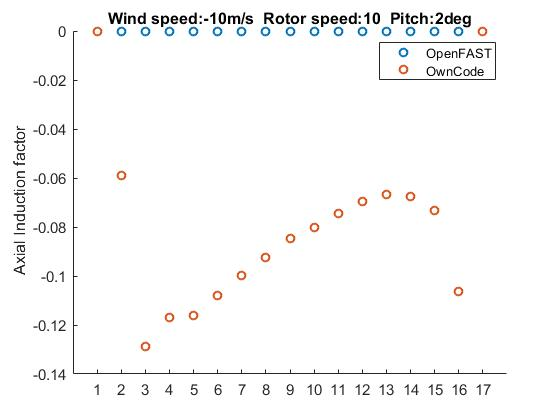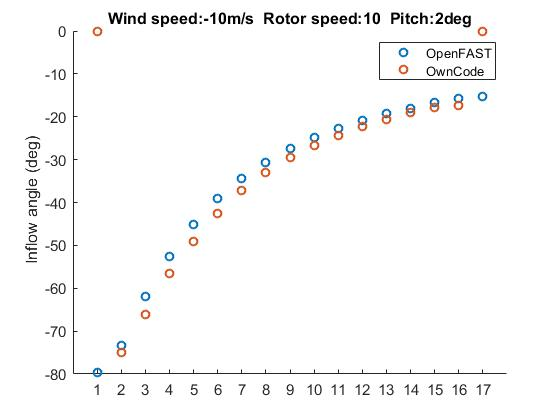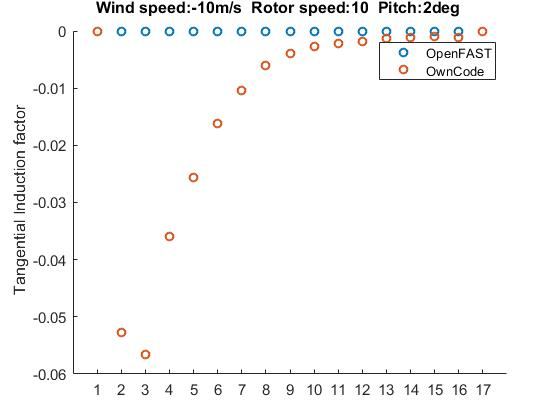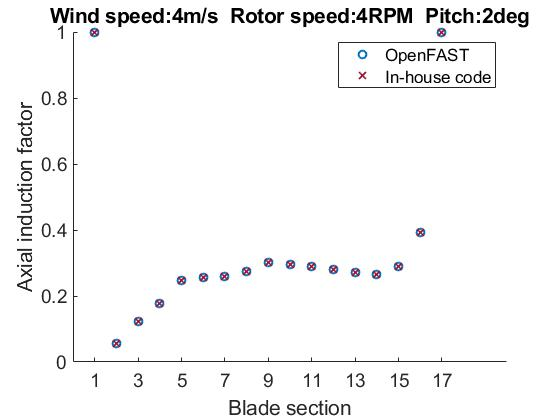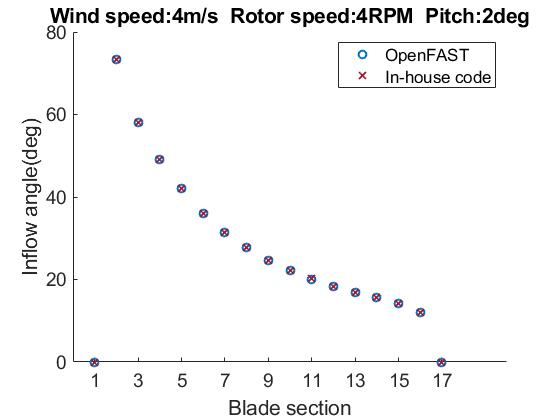Dear @Jason.Jonkman
To put it simply, in the cases where the Vx<0, Vy>0 why to hardcode Induction factors (a, a’), inflow angle (phi = atan(Vx, Vy). When solved such cases using the algorithm it is yielding solutions without convergence issues.

Regards,
Kumara

A couple points of clarification:

1. `phiIn` at the beginning of the call to `GetSolveRegionOrdering` is the value of `phi` from the previous step. Since the BEM equations may have multiple solutions, we use the region the previous solution was in to start searching regions.
2. The check for TSR < 2 was updated to use the absolute value of TSR, or `|TSR|<2`. See Bug fix: BEMT was disabled for negative inflow by bjonkman · Pull Request #1037 · OpenFAST/openfast · GitHub